﻿ Determination of the Fatigue Life of the Vehicle Construction Based on Strength CalculationsPublications are Open
Access in this journal
Article Versions
Export Article
• Normal Style
• MLA Style
• APA Style
• Chicago Style
Research Article
Open Access Peer-reviewed

### Determination of the Fatigue Life of the Vehicle Construction Based on Strength Calculations

American Journal of Mechanical Engineering. 2017, 5(6), 274-279. DOI: 10.12691/ajme-5-6-8
Published online: December 15, 2017

### Abstract

The article is divided into three parts. Except the foundations of theoretical knowledges about the fatigue life, the design of the breakdown truck body construction for a particular vehicle is also described in initials. In the second part the stress analysis of this construction is analyzed by finite element method (FEM). Based on this, at the end of the thesis, the fatigue life time is evaluated and compared with the experimental.

### 1. Introduction

With an increasing number of vehicles on the roads, the demand for breakdown trucks is rising. This article deals with the design of the breakdown truck body construction and its subsequent strength analysis, with a lifetime evaluation for piece or small-lot production. For the use in practice of such a breakdown truck, it is necessary to experimentally verify the fatigue life of the critical part of the body construction according to the EEC 55 standard. This must be done before the joining assembly. As a result, the idea of life prediction rises and she was based on numerical calculations, and critical design nodes could be optimized before a real fatigue test in the event of a negative result.

### 2. Basics of the Fatigue Life Time

The dependence of the stress amplitude on the number of cycles to the break is determined by the SN - curve constructed on the basis of a series of fatigue tests on samples of the same material, dimensions, shape and quality of the surface, subjected to cyclical loads with zero value of mean stress up to break. The individual samples are loaded descending from the highest load amplitude, with the observed parameter being the number of cycles to the fraction, to obtain a set of points corresponding to the number of loaded samples, which after the translation by curve will characterize the fatigue characteristic shown in Figure 1.

From the Figure 1 it is possible to reveal the dependence of the stress amplitude on the number of cycles to the fracture for which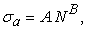(1)

one is more often written in shape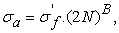(2)

where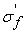is a coefficient of fatigue strength which is generally equal to the actual fracture stress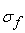which is higher than the ultimate strength itself.

• Figure 1. SN - curve construction for ussual low carbon steel

Low carbon steels are characterized by the fact that from the number of cycles 106 upwards, the amplitude of the stress does not decreasses, and therefore, under such a load, the component withstand theoretically an infinite number of cycles, without causing fatigue damage. From this assumption, a material property σc, which is called the material fatigue limit, was created. 1

The first step is to determine the amplitude of the symmetric cycle σar, which can be determined from each characteristic describing the relation between the normalized amplitude σa and the mean stress σm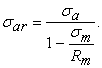(3)
• Figure 2. Cyclic load classification

(Stress range Rm = σmin / σmax )

In case of symmetric load, the mean stress σm = 0, so amplitude σar = σa. Thus, it is clear that each load cycle can be transformed into a symmetrical one. According to the relation (1), the relation for σar will be analogously derived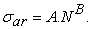(4)

By substitution equation (4) into (3) we get a relation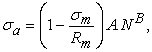(5)

respectively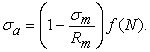(6)

Equation (6) represents a universal relation for determining the lifetime of components and constructions as it takes into account the influence of mean stress and hence the disproportion of load cycles. For the prediction of lifetime in case of the symetric stress response will be used the simplified equation (1) resp. (2). 2, 3

### 3. Design of the Breakdown Truck Body Construction

The priorities that need to be disscused in terms of designing the construction are as follows. From the practice it can be found that the approximate weight of the vehicle with the full construction is 2,3 tons, thus the permissible weight of the transported vehicle on the loading platform of the truck body construction will be 1,2 tons. For this purpose, it is necessary to reduce the weight of the structure to a minimum to ensure a higher transport capacity. However, this is only possible to a certain extent, since in practice these assemblies are very often overloaded above the permitted load.

The initial dimensions given by the manufactury (Figure 3) represent the arrangement of the fastening consols, which are suitable to be used. In this areas will be fastened transverse beams of the body construction, fastened by the bolt connections. It is also necessary to achieve the required dimensions of the body construction which is 5300 x 2100 mm. On the basis of geometric calculations, it was found that in order to ensure the angle of the required sloping platform (Figure 4) at the rear of the structure, it is necessary to cut part of the rear beams. The construction design created in the numerical environment (Figure 4) is made up of shell elements that represent the centerline areas of the used profiles. These elements have a number of advantages in front of 3D parts, especially in terms of time-based calculations.

• Figure 3. The main dimensions of the vehicle

The front part of the assembly contains a frame welded from rectangular profiles on which the fastening device is located which has a multiple aplications in fastening against displacement. A pair of U-shaped longitudinal reinforcing profiles, which are placed between the front bumper and the first transverse beam, serve as a supporting structure under the winding device. The longitudinal profiles, which are located along the entire length of both sides of the construction, fill the role of the guiding elements for the attachment of the cables to the side and rear lighting, which also have a reinforcing effect.

• Figure 4. Design of the breakdown truck body construction with detailed views of the critical nodes

It is assumed that the greatest stresses and deformations will arise in the area of attachment of the main beams of body construction to the main beams of the truck vehicle. Thus, two pairs of reinforcing elements are proposed here. They have an increased cross-sectional modulus in relation to the direction of force. They will be made of 5 mm thick sheet steel. These reinforcements are welded to the beams of body construction in locations of the holes and perimeter of the reinforcing parts. To the vehicle beams will be then fastened by bolt connections.

The second presumed critical node is the area of force aplication in the pulling attachment area, which is conected to a 12 mm steel plate. The force is transmitted thru the 6 mm thick profiles into the first center beam between the main beams of body construction, assuming a more favorable stress distribution during the load. It is need to be note, that the corners of the reinforcing plate at the point of contact with the bumper will act as stress concentrators, therefore will be fitted by chamfer. The uniform thickness of the rest profiles of the assembly will be 3 mm.

• Figure 5. Real breakdown truck body construction fixed on required vehicle for this particular case

### 4. Defining Conditions for Numerical Calculation

It is clear that the construction of Figure 4. will be made by welding the profiles. On this basis, the chosen material of the structural elements is steel EN ISO S355 JO, further it is structural, fine-grained, formable steel with guaranteed weldability (C ≤ 0,22%). Subsequently, based on the material data sheets, the physical properties of the material will be entered in the program environment.

The next step in defining the conditions is to divide the construction model into the final number of elements (creating mesh). It is necessary to take into account that the numerical calculation is crossing through each of these elements and it is therefore appropriate to consider the priority of the used parts in relation to the transfer of load, therefor the "finer" mesh will be used in more significant profiles in terms of time costs. In the less essential elements is leaved a "coarser" mesh . In both cases, there are used QUAD elements.

• Figure 6. "Finer" mesh in areas of expected increased stresses

Attachment of the breakdown truck body construction to the main beams of vehicle is simulated by the boundary conditions (Figure 7), (Figure 8.), which take the necessary degrees of freedom of movement to different parts of the model. The interaction between the individual elements in the assembly is defined by the bonds example of which could be seen in Figure 9.

• Figure 7. Boundary condition of the front part of the body construction
• Figure 8. Boundary condition of the rear section of the body construction

To simulate the body construction attachment on the main beams of the vehicle and and its fastening consoles red sections are created for removing the degree of freedom of movement in the Y-axis direction. The same approach is aplied for the areas of the bolt connections on the construction at the position of the fastening consoles, in which all degrees of freedom of movement are taken. In this way, the top of the construction will be secured against movement.

• Figure 9. Conections definition and boundary conditions for the rear part of the main beams of vehicle

Construction detail of the rear part of the truck body, which is shown in Figure 5, is assumed to carry the largest part of the load, so this area will be described detailed. The weld joint is defined by the bond, where the violet color represents the weld thickness and the red represents the contact surface. The boundary conditions described by the blue and orange arrows represents the positions and directions of the removed degrees of freedom of movement, specifically in the areas of the reinforcement elements holes where they were all taken.

The calculation of load effects is followed by EEC 55 standard, which deals with the approval of mechanical coupling parts of vehicles. Before calculation of the cyclic load force Fhs, it is first necessary to determine the reference horizontal force D between the trailer and the vehicle. Horizontal force is determined from the relation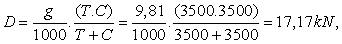where

D – horizontal force between trailer and vehicle [kN],

T – total weight of the vehicle [kg],

C – maximum total weight of the trailer [kg],

g – gravitational acceleration: 9,81 [m/s-2].

Subsequently the cyclic load force Fhs is determined by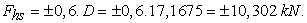An angle α = 15° ± 1° was determined according to the standard EEC 55, which indicates the direction of the load aplied on the towing device.

• Figure 10. The location, direction and magnitude of the cyclic force shown in the numerical model and in the real construction determined by standard EEC 55

For defining the location of the force,(the center of gravity of the sphere) was formed the reference point in which the components of the forces Fx and Fy were applied. 4, 5

### 5. Stress Analysis

The construction is analyzed on the basis of a static test and assessed from a strength point of view. The results of the analyzes are presented in the form of reduced stress fields according to HMH theory in MPa units. To highlight the differences between higher and lower stress areas, the upper limit of the scale was set to 70 MPa (red). Maximum stres response is marked in gray.

• Figure 11. Reduced stress fields during simulation of a static load test

The real lifetime test of the construction that was analyzed in the laboratory is shown in Figure 12. It is obvious that this construction does not correspond to a real, overall design proposal (Figure 5). It is a separate part of the assembly that is subject to the most degradation process, therefore the rest of the body construction will be neglected in case of spatial limitations of the test laboratory and also the time costs of the numerical calculation. 6, 7

• Figure 12. The field of the reduced stresses of the desired, critical part of the construction

From a comparison of static stress calculations, it is possible to see a stress increase from 133.28 MPa in the whole construction (Figure 11.) to 153.52 MPa in a separate critical part (Figure 12). This change is caused by altered boundary conditions. Figure 13 and Figure 14 shows a field of reduced stresses in critical areas obtained by numerical calculation.

• Figure 13. Fields of reduced stresses in critical areas by numerical calculation (area of attachment)
• Figure 14. Fields of reduced stresses in critical areas by numerical calculation (area of applied load)

A suitable optimization step for ensuring a longer fatigue lifetime by lowering the amplitude of stress is using of the Z-shaped optimization element in the joint of critical region shown in Figure 15.

• Figure 15. Z-shaped optimization element in the joint of critical region
• Figure 16. The result shape of the optimized construction design

Figure 16. shows the resulting breakdown truck body construction design, whose shape has been optimized based on the results of the stress analysis, to achieve the lowest weight.

### 6. Calculation of the fatigue Lifetime of the Construction

The estimated fatigue lifetime of the construction is determined from the relation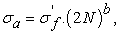from which N is expressed, corresponding to the number of cycles to the fracture at cyclic loading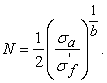Based on the fact that the stress response in relation to the load effect of the force has static, the alternating symmetrical waveform, therefore will be at the place of σa set value of the maximum reduced stress found in the static analysis, with the coefficients σf and b appointed on the material properties according to Table 1.

Thus, the number of cycles to fraction in the test will be calculated asThe result of the calculation is satisfying, because it is in the safe area of infinite fatigue lifetime of the material (Figure 1).The real test was performed in the laboratories of the VÚD in accordance with EEC 55 standard.

Used devices - electrohydraulic loader RSVH1,

- force sensor K & S LC-IE-20 kN- EK, number 010900, calibration certificate no. 0019 / 323.04 / 15.

Test was implemented on a test object signated TZ AGRI 1. In accordance with EEC 55 standard, the towing device was subjected to sinusoidal force with an amplitude from +10 302 N to -10 302 N at a frequency of 2,10 Hz. The number of cycles was 2x106.

### 7. Conclusion

Based on the strength analysis of the construction model, it was possible to determine the cyclic stress amplitude. It was then used in the calculation of fatigue lifetime prediction of the body construction by analytical relations. The constuction model has been judged as satisfying from the standpoint of simulated ECE 55 load. Based on this result bof the estimated number of cycles in the fatigue fraction, namely 4,0254.108 cycles, the result of the analytical solution could be verified in practice, a real fatigue test in the strength measurement laboratory. The evaluation report state the suitability of the design, and the actual test was stopped at the number of cycles 2,106, without permanent deformation, cracking or damage.

### Acknowledgments

This work was supported in part by the Ministry of Education of the Slovakia Foundation under grant projects VEGA No. 1/0731/16 and ITMS:26220120060 supported by the Research & Development Operational Programme funded by the ERDF.

### References

  Papacz, W., Tertel, E., Frankovský, P., & Kuryło, P. (2014). Analysis of the fatigue life of composite leaf springs. Applied Mechanics & Materials, (611). In article View Article  Tertel, E., Kuryło, P., & Cyganiuk, J. (2014). The distribution of stress in a sandwich conical shell panel under combined load. Procedia Engineering, 96, 467-476. In article View Article  Božek, P., & Turygin, Y. (2014). Measurement of the operating parameters and numerical analysis of the mechanical subsystem. Measurement Science Review, 14(4), 198-203. In article View Article  Trebuňa, F., Šimčák, F. (2004). Odolnosť prvkov mechanických sústav. TUKE. In article  Frankovský, P., Kicko, M. (2017). Strength analysis and the fatigue life estimation of breakdown truck body construction, TUKE. In article PubMed  Vaško, M., & Sága, M. (2013). Application of fuzzy structural analysis for damage prediction considering uncertain S/N curve. In Applied Mechanics and Materials (Vol. 420, pp. 21-29). Trans Tech Publications. In article View Article  Vavro, J., Kováčiková, P., Kopas, P., & Handrik, M. (2014). Simulation and analysis of defect distribution in passenger car tire under dynamic loading. In Applied mechanics and materials (Vol. 611, pp. 544-547). Trans Tech Publications. In article View ArticleThis work is licensed under a Creative Commons Attribution 4.0 International License. To view a copy of this license, visit http://creativecommons.org/licenses/by/4.0/